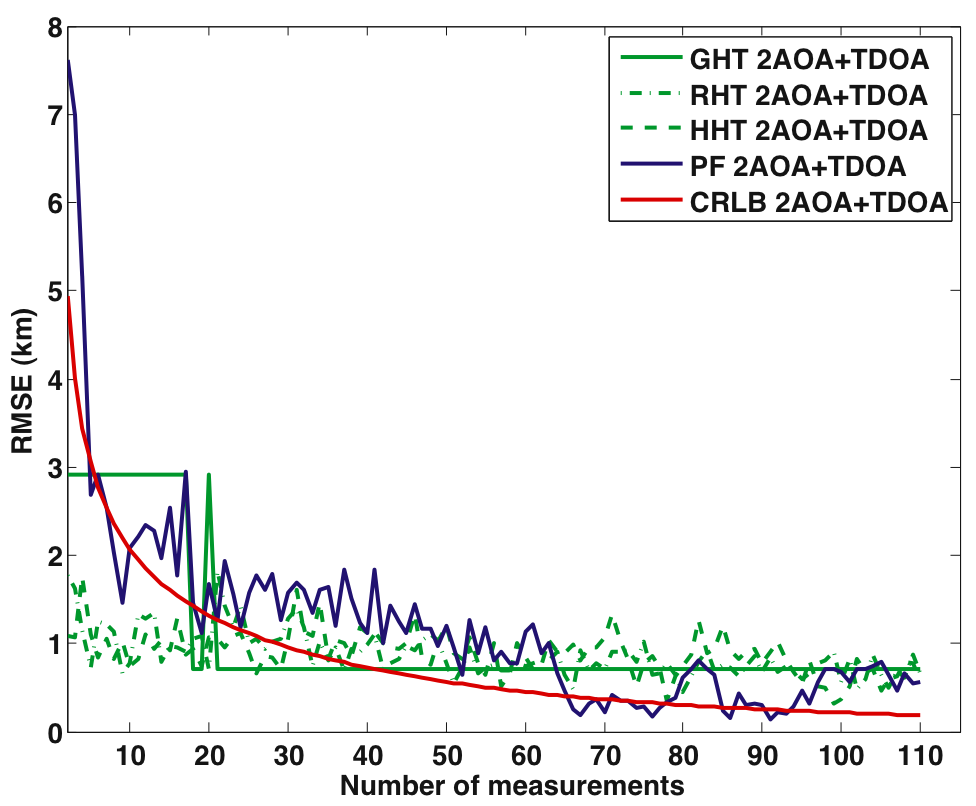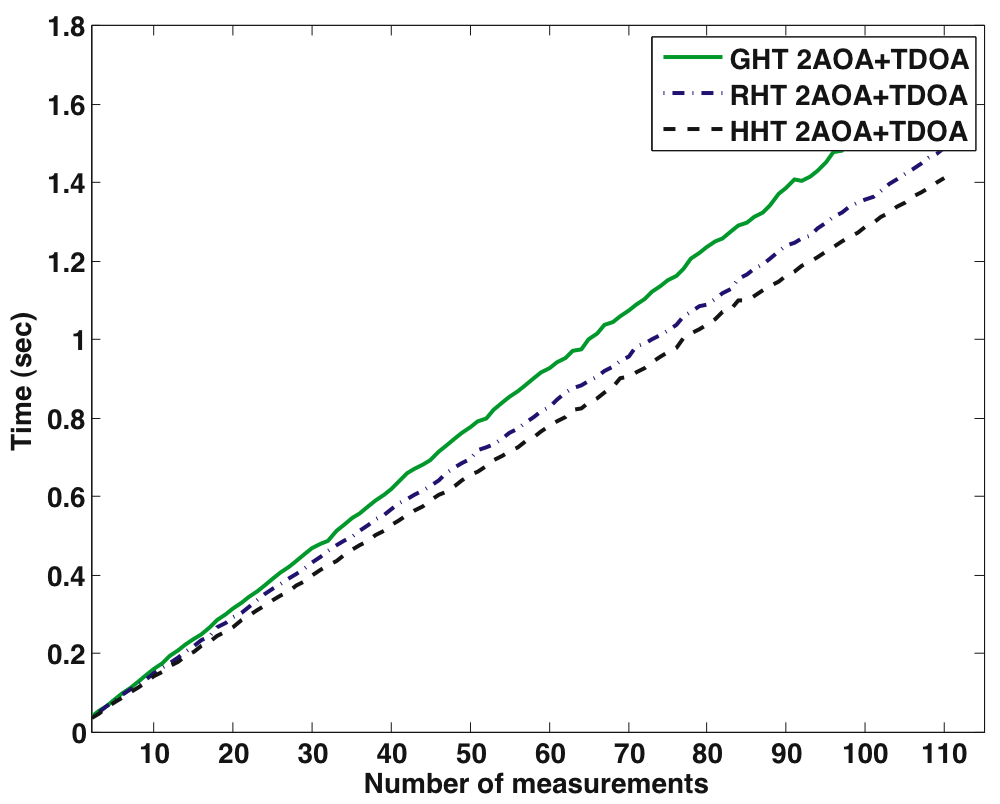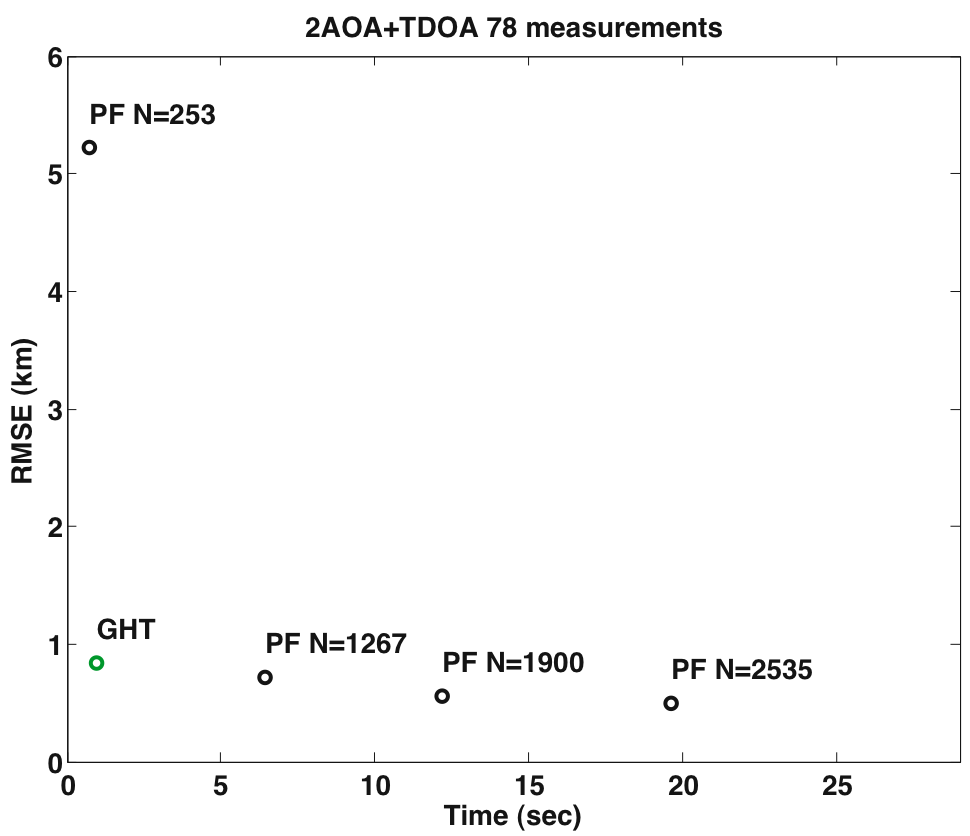Date Tue 24 August 2010 Tags science

In computer science it is common to measure algorithms performance by using O notation. Every book on algorithms development will start from this topic and although it is still good rule of thumb, the practical value of O-notation now becoming obsolete.

For example Mathworks dropped Flops since version 6, the command which was a good indicator of number of floating points operations in second.

For another example see Is multiplication slower then addition,

where Prof Daniel Lemire after perfoming basic tests concludes that "Hence, simple computational cost models (such as counting the number of multiplications) may not hold on modern superscalar processors."

During my PhD thesis development, I compared different version Particle filter - stochastic algorithm, which performance depends on number of particles used with Hough Transform based algorithms, which performance depends on a size of the grid in accumulator. In this post, I will show how Pareto optimal points can be used as good visualisation techniques for algorithms comparison.

If you come from computer science background you are very unlikely have been exposed to Pareto front, but it is very well developed and used in Evolutionary Computation and Operations Research.

Definition: We say that a vector of decision variables $\hat x^{*} \in F$ is Pareto optimal if there does not exist another $\hat x \in F$ such that $f(\hat x) \le f(\hat x^{*})$ for all $i = 1, \hdots k$ and $f_{j}(\hat x)< f_{j}(\hat x)$ for at least one j. In words, this deﬁnition says that $\hat x^{*}$ is Pareto optimal if there exists no feasible vector of decision variables $\hat x^{*} \in F$ which would decrease some criterion without causing a simultaneous increase in at least one other criterion. Unfortunately, this concept almost always gives not a single solution, but rather a set of solutions called the Pareto optimal set. The vectors $\hat x^{*}$ corresponding to the solutions included in the Pareto optimal set are called nondominated. The plot of the objective functions whose nondominated vectors are in the Pareto optimal set is called the Pareto front.

There are many way to plot Pareto front and some of the basic Pareto fronts can be found on EMOO webpage. I have come across really amazing Pareto front of knapsack problem, but I can't find it right now.

Now, in modern algorithms like particle filter, genetic algorithm or grid based method, the performance (accuracy) of the algorithm depends on number of particles, size of the population or step size of the grid.

My usual plot for algorithms comparison in terms of accuracy against number of measurements looks like this:Comparison on terms of RMS against number of measurements

with corresponding timeHough Transform variants running time against number of measurement

Now let's say we fix our model on 78 measurements (time of the algorithms are not too extreme) and plot points extracted from Pareto font.Pareto optimal points for GHT and PF

Basically Pareto front can be viewed as additional dimension to [Comparison on terms of RMS against number of measurements] plot, but in such way it is clear and concise representation of algorithms comparison. Of cause for statistical validity, the simulation had to run hundred times and average time over hundred runs taken. Another drawback that all algorithms have to be performed on same computer with same configuration, which limits use of Amazon EC2 for example.